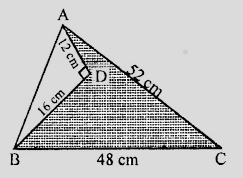"
">

# Find the area of the shaded region in figure."

To do:

We have to find the area of the shaded region in the figure.

Solution:

From the figure,

In $\triangle ABC$,

$AC = 52\ cm, BC = 48\ cm$

In right angle triangle $ADC$,

$\angle D = 90^o, AD = 12\ cm, BD = 16\ cm$

By Pythagoras theorem,

$AB^2=AD^2 + BD^2$

$=(12)^2 + (16)^2$

$= 144 + 256$

$= 400$

$= (20)^2$

$\Rightarrow AB = 20\ cm$

Area of the $\triangle \mathrm{ABD}=\frac{1}{2} \times \mathrm{AD} \times \mathrm{BD}$

$=\frac{1}{2} \times 12 \times 16$

$=96 \mathrm{~cm}^{2}$

Area of $\triangle \mathrm{ABC}$ with sides $52 \mathrm{~cm}, 48 \mathrm{cm}, 20 \mathrm{~cm}$ is,

$s=\frac{a+b+c}{2}$

$=\frac{52+48+20}{2}$

$=\frac{120}{2}$

$=60$

Area of the triangle $=\sqrt{s(s-a)(s-b)(s-c)}$

$=\sqrt{60(60-52)(60-48)(62-20)}$

$=\sqrt{60 \times 8 \times 12 \times 40}$

$=\sqrt{10 \times 2 \times 3 \times 2 \times 2 \times 2 \times 2 \times 2 \times 3 \times 10 \times 2 \times 2}$

$=10 \times 2 \times 2 \times 2 \times 2 \times 3$

$=480 \mathrm{~cm}^{2}$

Area of the shaded portion $=480-96$

$=384 \mathrm{~cm}^{2}$

Updated on: 10-Oct-2022

34 Views